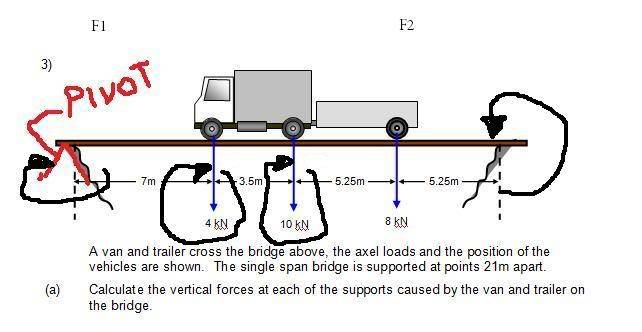# Calculate the tension in the string

Doc Al
Mentor
For the sum of the clockwise torques I understand that 200*L+200(L/2) but I don't understand 200*(3L/2) where did the "3L" come from ?
L + L/2 = 3L/2
You can also write that as: (3/2)*L

You can also do this:
200*L + 200*(L/2) = 200*L + 100*L = 300*L

Please convince yourself that these are equivalent.

L + L/2 = 3L/2
You can also write that as: (3/2)*L

You can also do this:
200*L + 200*(L/2) = 200*L + 100*L = 300*L

Please convince yourself that these are equivalent.

Ah yes I see if we replace L with 1 we get 1.5=1.5 meaning the formula becomes 300*L=L*T*Sin30 meaning T=600N.

The next question im stuck on is http://img63.imageshack.us/img63/4524/yhse1.th.jpg [Broken]

I would think the answer is 300*L=L*T*Sin50 .

Last edited by a moderator:
Doc Al
Mentor
I would think the answer is 300*L=L*T*Sin50 .
The left-hand side (which represents clockwise torques) is correct--it's the same as the last problem. But the right-hand side is not correct. The angle is not the only thing that changes: the distance to the pivot point also changes--it's not L anymore.

The left-hand side (which represents clockwise torques) is correct--it's the same as the last problem. But the right-hand side is not correct. The angle is not the only thing that changes: the distance to the pivot point also changes--it's not L anymore.

So this would make it 300*L=L/2*T*Sin30 ?

Doc Al
Mentor
So this would make it 300*L=L/2*T*Sin30 ?
You now have the correct distance on the right-hand side (L/2), but what happened to the angle?

You now have the correct distance on the right-hand side (L/2), but what happened to the angle?

Sorry about that I meant Sin50.

Doc Al
Mentor
Sorry about that I meant Sin50.
Good! (I thought so.) Now you can solve for the tension--once again, the actual value of L doesn't matter.

Good! (I thought so.)
Right so the equation now becomes 300*L=L/2*T*Sin50.

Doc Al
Mentor
Right so the equation now becomes 300*L=L/2*T*Sin50.
Yep.

Doc Al
Mentor
So if I replace L with 2 I get T=600/sin50 meaning T=783.
Correct. But realize you don't have to "replace" L with any number--the L just drops out:
300*L=L/2*T*Sin50 becomes 300=1/2*T*Sin50 if you cancel the L on both sides. (Which means dividing both sides by L.)

Now im stuck with the following question ...
Another equilibrium problem. What are the conditions for equilibrium? Forces must add to zero and torques about any point must add to zero. Label the vertical support forces (at each end of the bridge) F1 & F2. Hint: A good point to use as a pivot is either end of the bridge.

Another equilibrium problem. What are the conditions for equilibrium? Forces must add to zero and torques about any point must add to zero. Label the vertical support forces (at each end of the bridge) F1 & F2. Hint: A good point to use as a pivot is either end of the bridge.

Alright so if I lable the force between 4kN and 10kN F1 and from 10kN to 8kN F2 and place an pivot at the left hand side of the bridge would this be correct and what would the equation be for the clockwise rotation= counter clockwise rotation ?

As seen below
http://img211.imageshack.us/img211/5756/klcw7.th.jpg [Broken]

Last edited by a moderator:
Doc Al
Mentor
Alright so if I lable the force between 4kN and 10kN F1 and from 10kN to 8kN F2 and place an pivot at the left hand side of the bridge would this be correct and what would the equation be for the clockwise rotation= counter clockwise rotation ?
No. F1 is the upward force of the support on the left end of the bridge, F2 is the upward force on the right end of the bridge.

If you pick the left side of the bridge as your pivot, you will have the three axial loads producing the clockwise torques and the force F2 producing the counter-clockwise torques. Find those torques just like in all the previous problems.

Would it be *F1 14kN*10.5M=8kN*5.25M*F2 ? am I correct ?

Last edited:
Doc Al
Mentor
Would it be *F1 14kN*10.5M=8kN*5.25M*F2 ? am I correct ?
No. There are three forces (each at a different distance from the pivot) that create clockwise torques. And the force F2, which creates the counter-clockwise torque, is how far from the pivot?

I encourage you to study the examples discussed on the webpage I linked in post #37.

No. There are three forces (each at a different distance from the pivot) that create clockwise torques. And the force F2, which creates the counter-clockwise torque, is how far from the pivot?

I encourage you to study the examples discussed on the webpage I linked in post #37.

Alright so is this correct ?Meaning that ths distance from the pivot is 5.25M+5.25M?

Doc Al
Mentor
No, the distance of F2 from the left pivot point is: 7m + 3.5m + 5.25m + 5.25m = 21m. (You didn't even have to do that addition: the problem states that the bridge supports are 21m apart.)

No, the distance of F2 from the left pivot point is: 7m + 3.5m + 5.25m + 5.25m = 21m. (You didn't even have to do that addition: the problem states that the bridge supports are 21m apart.)

I think the total Clockwise force F1 would be equal to 22kN*21m=462 correct but what would the counter clockwise force be then?

Last edited: